## General Question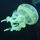# What is the formula for the volume of a square pyramid?

Asked by pcmonkey (427) March 9th, 2011

It’s V=⅓Bh but what does B=? pi X r2? I was thinking that but I thought that the pi x r2 thing was for a circle also so im not sure. Please help.

Observing members: 0Composing members: 0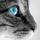It’s V=⅓b(sq)h or Volume equals one third (base squared) times height

I think you are confusing some of a cone volume formula

optimisticpessimist (3909)“Great Answer” (4) Flag as…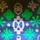I think you may be under the misconception that B always equals pi x r(sq). B is equal to the base, not a specific formula.

2CDenzy (442)“Great Answer” (2) Flag as…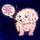⅓ (area of base)x(height)

Allie (17436)“Great Answer” (2) Flag as…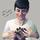pi*r^2 is the formula for area of a circle. B means area of the base in general for any formula. Since your base is a square in this case, B = s^2 where s is the length of one side of the square. @optimisticpessimist provided the correct formula.

Mariah (25831)“Great Answer” (2) Flag as…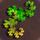V = ⅓*B*H is the volume of any pyramid, given a base with area B and the pyramid’s height H. The volume of any pyramid is ⅓ that of a prism with the same base and height.

For a rectangular pyramid, Base = Length*Width. For a square pyramid, this reduces to Base = Side^2. The volume of a square pyramid is Volume = ⅓*Side^2*Height.

2*Pi*R is the formula for the circumference of a circle, and Pi*R^2 is the formula for the area of a circle. The second formula, the area of a circle, is used to find the base of a cone. The equation for the volume of a cone, or a circular pyramid, is V = ⅓*Pi*R^2*H

PhiNotPi (12670)“Great Answer” (3) Flag as…Ah yeah, the base isn’t squared, so @Allie‘s formula is correct.

Mariah (25831)“Great Answer” (0) Flag as…Part of the confusion is that there is no name for the shapes that would include cones and pyramids and which all have the same volume formula of ⅓ Bh, where B is the area of the base. By contrast, the name prism includes both cylinders and rectangular solids.

LostInParadise (25507)“Great Answer” (0) Flag as…or